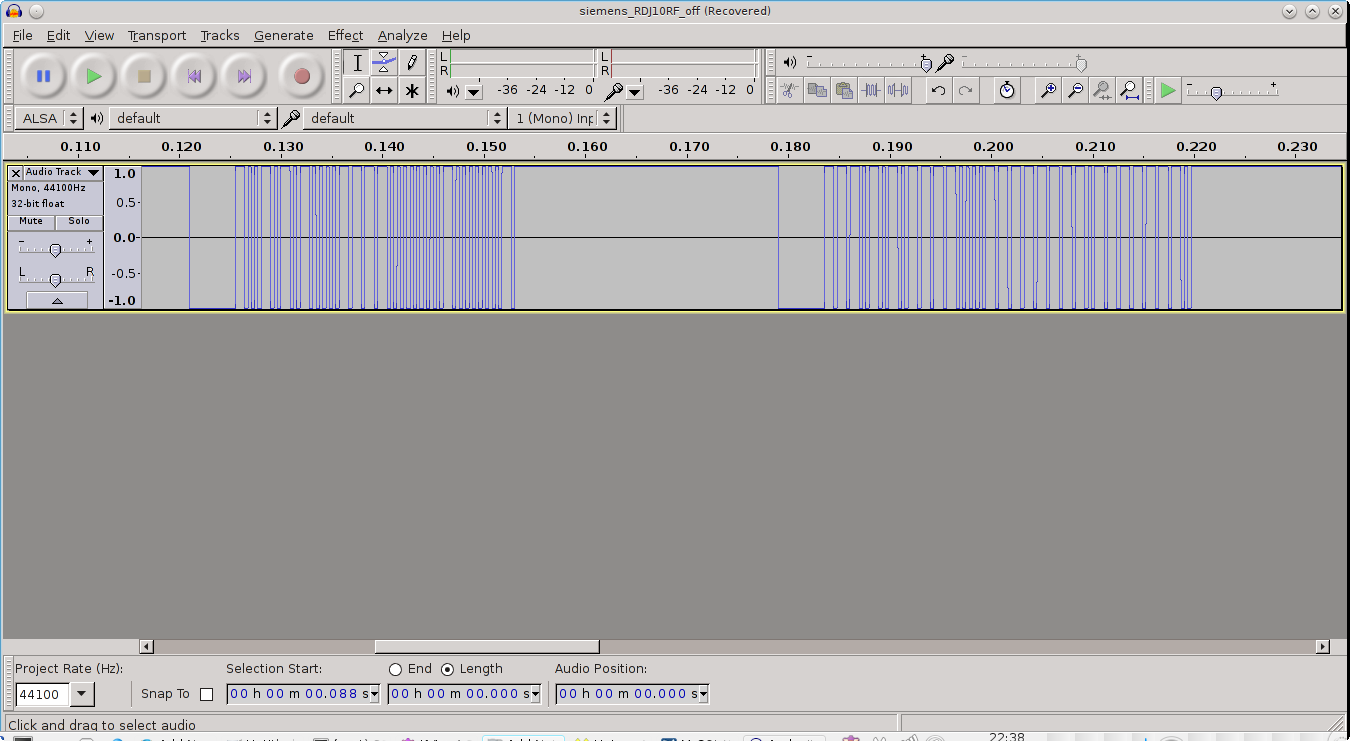# Heating control rcr10/433-GB RDJ10RF siemens

At the bottom of this post is the Arduino sketch you can use with the IRremote.h library. This library is used to send infrared light used on remote controls for television and video recorders and such. Interestingly the Siemens radio heating control uses the same encoding for its radio signal.

The structure of the radio signal is thus.There are two sets of pulses. The first of the left is 32 bits is Infrared Sony encoded information. This is explained further here. The 32 + 32 bit data is sent 4 times in a row. I guess for redundancy and reliability. I found also if its only sent once it does not work. So 64 bits x 4 pulses are necessary.

http://www.righto.com/2010/03/understanding-sony-ir-remote-codes-lirc.html

the library IRremote does have an API sendSONY but I found it does not work for my purposes here, because the frequency passed in the library did not fit my frequency. So I used the SendRaw() function. I also defined my own one bit and zero bit and repeated them according to the array.

I have noticed the first set of 32 bits is always the same and it related to the second set. Its related in a way that is quite interesting. Its the original 32 bits but inverted (not gated).

I have found its inverted except for 2 bits. Bits 9 and 32. This bit inversion defines whether the signal being sent is on or off. You can see this from my code below.

To get this code working on your own heater. You probably will need to change the bits that are sent here and there. As they are all unique for “security” sake. There are also dip switched to change the identity. You can record the signal from your timer with a 433 Mhz receiver via your sound card. Then interpret the ones and zeros for off and put it in the heating_code_second array. This stores the off signal. Then the array inverts the necessary bits to make the signal on. The whole command for the Arduino code accepts a 1 for on and 0 for off over the serial link.

To interface the Arduino to the radio one needs a 433Mhz transmitter and  receiver pair. The receiver can be used to record the signal from the original siemens transmitter into audacity. Then run the sketch below to check it matches your out put.```/*
* IRremote: IRsendDemo - demonstrates sending IR codes with IRsend
* An IR LED/radio transmitter must be connected to Arduino PWM pin 3.
* Version 0.1 July, 2009
* http://arcfn.com
*/

#include <IRremote.h>

IRsend irsend;

// just added my own array for the raw signal
unsigned int digitalOne = {0,820,370,0};
unsigned int digitalZero = {0,200,370,0};
//off
unsigned int heating_code_first;
const unsigned int heating_code_second =
{1,0,0,1,0,1,0,1,0,1,0,0,1,1,1,1,0,0,0,0,0,0,0,0,1,0,0,0,0,0,0,0,0};
String inputString = "";         // a string to hold incoming data
boolean stringComplete = false;  // whether the string is completevoid
setup() {
Serial.begin(9600);
}

void loop() {// altered the code just to send/test my raw code
if (stringComplete) {
Serial.println(inputString);
// clear the string:
unsigned int input_num = inputString.toInt();
inputString = "";
stringComplete = false;
unsigned int i=0;
for (i=0;i < 33;i++) {
heating_code_first[i] = heating_code_second[i];
}
if (input_num == 1) {//heating off
//Serial.write("Heating on bit inversion");
if (heating_code_first == 0) {
heating_code_first =1;
} else {
heating_code_first =0;
}
if (heating_code_first == 0) {
heating_code_first =1;
} else {
heating_code_first =0;
}
}
unsigned int pulse_count=0;
for (pulse_count=0;pulse_count < 4;pulse_count++) {
int bit_count =0;
for (bit_count=0;bit_count < 33;bit_count++) {
if (heating_code_first[bit_count] == 1) {
irsend.sendRaw(digitalOne,4,40);
} else {
irsend.sendRaw(digitalZero,4,40);
}
}

delay(28);//Big delay between two pulses.
for (bit_count=0;bit_count < 33;bit_count++) {
if (heating_code_first[bit_count] == 0) {
irsend.sendRaw(digitalOne,4,40);
} else {
irsend.sendRaw(digitalZero,4,40);
}
}
delay(15);
}

//Serial.println("Done");
}

//delay(2000);
serialEvent();
}//close main loop

void serialEvent() {
while (Serial.available()) {
// get the new byte: